# Nonlinear Optical Interactions

This is a continuation from the previous tutorial - nonlinear optical susceptibilities.

Optical susceptibilities in the frequency domain are, in general, complex quantities. Following the same convention, used in the linear optical susceptibility tutorial and the material dispersion tutorial for complex linear susceptibility in the frequency domain, complex nonlinear susceptibilities in the frequency domain can be expressed as $$\boldsymbol{\chi}^{(2)}=\boldsymbol{\chi}^{(2)'}+\text{i}\boldsymbol{\chi}^{(2)''}$$ and $$\boldsymbol{\chi}^{(3)}=\boldsymbol{\chi}^{(3)'}+\text{i}\boldsymbol{\chi}^{(3)''}$$ to define their real and imaginary parts clearly.

Similarly to the case of linear susceptibility discussed in the material dispersion tutorial, the imaginary part of a nonlinear susceptibility is always associated with the intrinsic resonances of a material. Such resonances signify the transitions between different energy levels of the material. Near the resonance frequencies that are relevant to a particular susceptibility, the imaginary part of the susceptibility peaks while the real part displays a highly dispersive behavior.

We shall not get into discussions on the quantum-mechanical characteristics of nonlinear susceptibilities as a function of energy levels of a material, or on the intrinsic relationship between the real and imaginary parts of a nonlinear susceptibility. Such subjects are of special interest in studies of material properties as well as in the application of nonlinear optics as a spectroscopic tool.

For our purpose in these tutorials, it suffices to understand that the imaginary part of a nonlinear susceptibility is strongly coupled to the transition resonances of a material in a way similar to the dependence of the imaginary part of the linear susceptibility on the material resonances. In addition, the real and imaginary parts of a nonlinear susceptibility are also related in a way similar to the relationship, expressed in the Kramers-Kronig relation, between those of the linear susceptibility.

An optical interaction that is characterized by a real frequency-dependent susceptibility, or the real part of a complex susceptibility, is generally classified as parametric, whereas one that is associated with the imaginary part of a complex frequency-dependent susceptibility is nonparametric.

In a nonparametric process, the state of the material changes, and the total optical energy also changes accordingly, because the process is connected to resonant transitions in the material. In a purely parametric process, however, both the state of the material in the medium and the total optical energy remain unchanged because the process does not cause any net exchange of energy between the optical field and the medium.

In a parametric linear process, the energy of any given optical frequency component is conserved because a linear process does not couple fields of different frequencies, as can be seen from the fact that linear susceptibility $$\boldsymbol{\chi}^{(1)}(\omega)$$ is a function of a single frequency.

In a parametric nonlinear process, energy exchange among different frequency components caused by nonlinear coupling among them usually occurs though the sum of energies from all of the interacting frequency components is conserved. For example, in a parametric process characterized by a real $$\boldsymbol{\chi}^{(2)}(\omega_3=\omega_1+\omega_2)$$, optical energy can be transferred from the frequency components at $$\omega_1$$ and $$\omega_2$$ to the component at $$\omega_3$$, or vice versa. Therefore, the energy in each individual frequency component may change, but the total optical energy contained in all three frequency components is conserved because there is no net exchange of energy between the optical field and the medium in a parametric process.

There are two features that are unique to nonlinear optical processes: one is optical frequency conversion, and the other is field-dependent modification of a certain material property. All nonlinear processes exhibit at least one, though not necessarily both, of these two features. All functional nonlinear optical devices take advantage of one or both of these two unique features.

As we have seen again and again in early tutorials, a very important condition for efficient coupling among optical waves or among optical modes is phase matching, regardless of which physical mechanism is responsible for the coupling. Phase matching is also most important for efficient nonlinear optical interactions. The phase-matching condition for a second-order nonlinear interaction that is characterized by the relation $$\omega_3=\omega_1+\omega_2$$ among the interacting frequencies is

$\tag{9-41}\mathbf{k}_3=\mathbf{k}_1+\mathbf{k}_2$

The phase-matching condition for a third-order interaction that is characterized by the relation $$\omega_4=\omega_1+\omega_2+\omega_3$$ among the interacting frequencies is

$\tag{9-42}\mathbf{k}_4=\mathbf{k}_1+\mathbf{k}_2+\mathbf{k}_3$

When a particular frequency changes sign, the corresponding wavevector in the phase-matching condition also changes sign.

The phase-matching condition is not automatically satisfied in a parametric process that leads to the conversion of optical frequencies. However, it is automatically satisfied in a parametric process that does not convert energy from one optical frequency to another. It is also automatically satisfied in any nonparametric process where an exchange of energy between the optical field and the medium occurs.

Second-order nonlinear optical processes

Table 9-4 below lists the second-order nonlinear processes. As indicated in this table, the contributing susceptibility for each of these processes is the real part, $${\boldsymbol{\chi}^{(2)}}'$$, of a frequency-dependent second-order susceptibility. Therefore, all of them are parametric in nature, and the frequencies involved in a second-order process of interest are generally far away from any resonance frequencies of the nonlinear medium.

Although each process listed in Table 9-4 demonstrates a unique phenomenon and has its own specific applications, all of them are basically parametric frequency conversion processes generally characterized by a real susceptibility of the form $$\boldsymbol{\chi}^{(2)}(\omega_3=\omega_1+\omega_2)$$. The differences among different processes come either from different experimental conditions or from different subjective purposes of application.

The full permutation symmetry expressed in (9-28) [refer to the nonlinear optical susceptibilities tutorial] applies to the real susceptibilities characterizing parametric second-order processes. Therefore, the processes of sum-frequency generation, difference-frequency generation, and optical parametric generation listed in Table 9-4 have the same nonlinear susceptibility.

In sum-frequency generation, shown in Figure 9-1(a) below, an optical wave at a high frequency, $$\omega_3$$, is generated through nonlinear interaction of optical waves at two lower frequencies, $$\omega_1$$ and $$\omega_2$$, with the nonlinear medium.

Second-harmonic generation is the degenerate case of sum-frequency generation for $$\omega_1=\omega_2=\omega$$ and $$\omega_3=2\omega$$, as shown in Figure 9-1(b) below.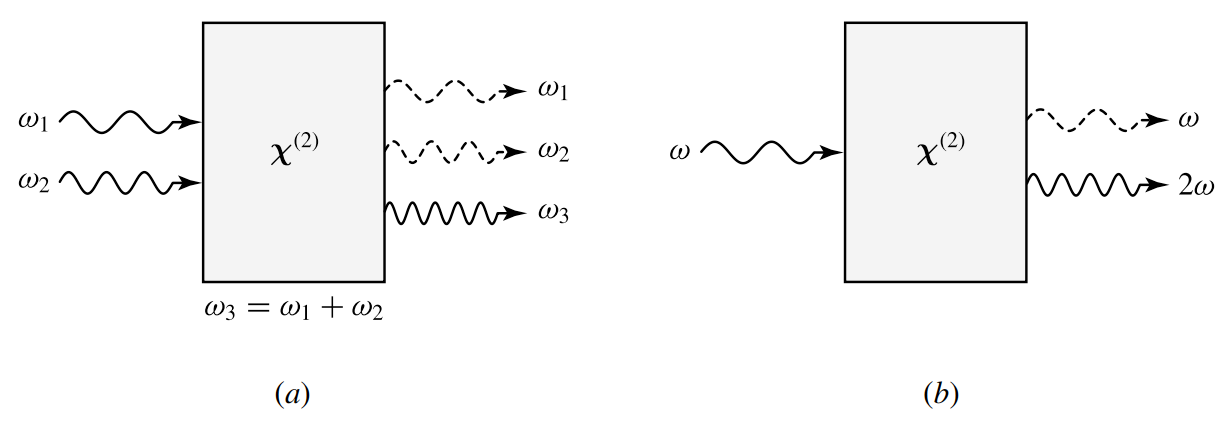Figure 9-1. (a) Sum-frequency generation and (b) second-harmonic generation. Second-harmonic generation is the degenerate case of sum-frequency generation.

In difference-frequency generation, shown in Figure 9-2(a) below, two optical waves at frequencies $$\omega_3$$ and $$\omega_1$$ interact with the nonlinear medium to generate an optical wave at the difference frequency $$\omega_2=\omega_3-\omega_1$$.

Optical rectification, shown in Figure 9-2(b) below, is the degenerate case of difference-frequency generation for $$\omega_3=\omega_1=\omega$$ and $$\omega_2=0$$.

In nondegenerate sum- and difference-frequency generation, two optical waves at different frequencies have to be supplied at the input. In second-harmonic generation and optical rectification, only one optical wave is needed at the input.

Second-harmonic generation performs the function of frequency doubling for the input optical wave, whereas optical rectification generates a DC electric field in a nonlinear medium through the interaction of an optical wave with the medium.

In sum-frequency generation, energy conservation implied by the relation $$\omega_3=\omega_1+\omega_2$$ requires that one photon at $$\omega_1$$ and another at $$\omega_2$$ be annihilated simultaneously when one photon at $$\omega_3$$ is generated. The intensities of both input waves at $$\omega_1$$ and $$\omega_2$$ decrease as the intensity of the output wave at $$\omega_3$$ grows.

In difference-frequency generation characterized by the relation $$\omega_2=\omega_3-\omega_1$$, however, one photon at the input frequency $$\omega_1$$ is generated simultaneously with one photon at the difference frequency $$\omega_2$$ when one photon at $$\omega_3$$ is annihilated. Therefore, as the intensity of the output wave at $$\omega_2$$ grows, the intensity of the low-frequency input wave at $$\omega_1$$ also increases though that of the high-frequency input wave at $$\omega_3$$ diminishes.

The reverse process of sum-frequency generation is optical parametric generation, in which an optical wave at a high frequency $$\omega_3$$ interacts with the nonlinear medium and generates two optical waves at the lower frequencies $$\omega_1$$ and $$\omega_2$$, as shown in Figure 9-3 below.

In this process, one photon at $$\omega_3$$ is annihilated when two photons at $$\omega_1$$ and $$\omega_2$$, respectively, are generated. This process can occur spontaneously in the form of parametric fluorescence. In practical applications, it normally takes place with a feedback or with another input wave at either $$\omega_1$$ or $$\omega_2$$. When a feedback is provided, usually by placing the nonlinear crystal in a resonant optical cavity, optical parametric oscillation is possible with only one input wave at $$\omega_3$$. If an input wave at one of the parametric frequencies, say $$\omega_1$$, is also provided, the process is basically the same as that of difference-frequency generation except that the purpose is now the amplification of the signal at the input frequency $$\omega_1$$ rather than the generation of the wave at the difference frequency $$\omega_2$$. For this reason, this process is called optical parametric amplification.

The special case of the Pockels effect can also be considered as parametric mixing of an optical field at $$\omega$$ with a DC or low-frequency electric field though it is generally described in terms of a modification on the permittivity tensor of a crystal by the DC or low-frequency electric field.

Example 9-4

Starting with a Nd : YAG laser that emits at a single wavelength of 1.064 μm, what different optical wavelengths can possibly be generated through second-order nonlinear optical processes in a single step? What can be generated in two cascaded steps?

Starting from $$\lambda_\omega=1.064$$ μm, we can generate its second harmonic at $$\lambda_{2\omega}=\lambda_\omega/2=532\text{ nm}$$ by frequency doubling in one step through $$\boldsymbol{\chi}^{(2)}(2\omega=\omega+\omega)$$ in a nonlinear crystal. We can also use optical parametric generation to generate pairs of tunable wavelengths at $$\lambda_1,\lambda_2\gt1.064$$ μm for $$1/\lambda_1+1/\lambda_2=1/\lambda_\omega$$ in one step through $$\boldsymbol{\chi}^{(2)}(\omega=\omega_1+\omega_2)$$ in a nonlinear crystal. Therefore, it is possible to obtain visible wavelength at 532 nm and a range of tunable infrared wavelengths longer than 1.064 μm.

In two cascaded steps, many more wavelengths can be generated. The second harmonic can be further frequency doubled to the fourth harmonic at $$\lambda_{4\omega}=\lambda_\omega/4=266$$ nm. We can also mix the fundamental and the second harmonic in a nonlinear crystal to generate the third harmonic at $$\lambda_{3\omega}=\lambda_\omega/3=354.7$$ nm by sum-frequency generation through $$\boldsymbol{\chi}^{(2)}(3\omega=\omega+2\omega)$$ in a nonlinear crystal. The second harmonic at 532 nm generated in the first step can be used to generate a range of tunable wavelengths longer than 532 nm through optical parametric generation. This range of tunable wavelengths longer than 532 nm can also be covered by frequency doubling the tunable infrared wavelengths generated through optical parametric generation in the first step, as well as by mixing the tunable infrared wavelengths with the fundamental at 1.064 μm through sum-frequency generation.

We can see further along this line that if we take merely three steps of second-order nonlinear optical mixing processes, either all in cascade or mixed in parallel/cascade, it is possible to cover a wide spectral range from the deep ultraviolet to the far infrared by starting with a single laser wavelength. The requirements for these processes to take place efficiently, as well as their limitations, are discussed in following tutorials.

Third-order nonlinear optical processes

The third-order nonlinear processes of common interest are listed in Table 9-5 below. Among these third-order processes, some, such as third-harmonic generation, parametric frequency conversion, and the optical Kerr effect, are parametric, whereas others, such as absorption saturation, and stimulated Raman scattering, are nonparametric. As can be seen in Table 9-5, the contributing susceptibility of a parametric third-order process is the real part, $${\boldsymbol{\chi}^{(3)}}'$$, and that of a nonparametric process is the imaginary part, $${\boldsymbol{\chi}^{(3)}}''$$, of a frequency-dependent third-order susceptibility.

As many as four different optical frequencies can participate in a third-order parametric frequency conversion process. As shown in Figure 9-4 below, there are a few different variations of this process.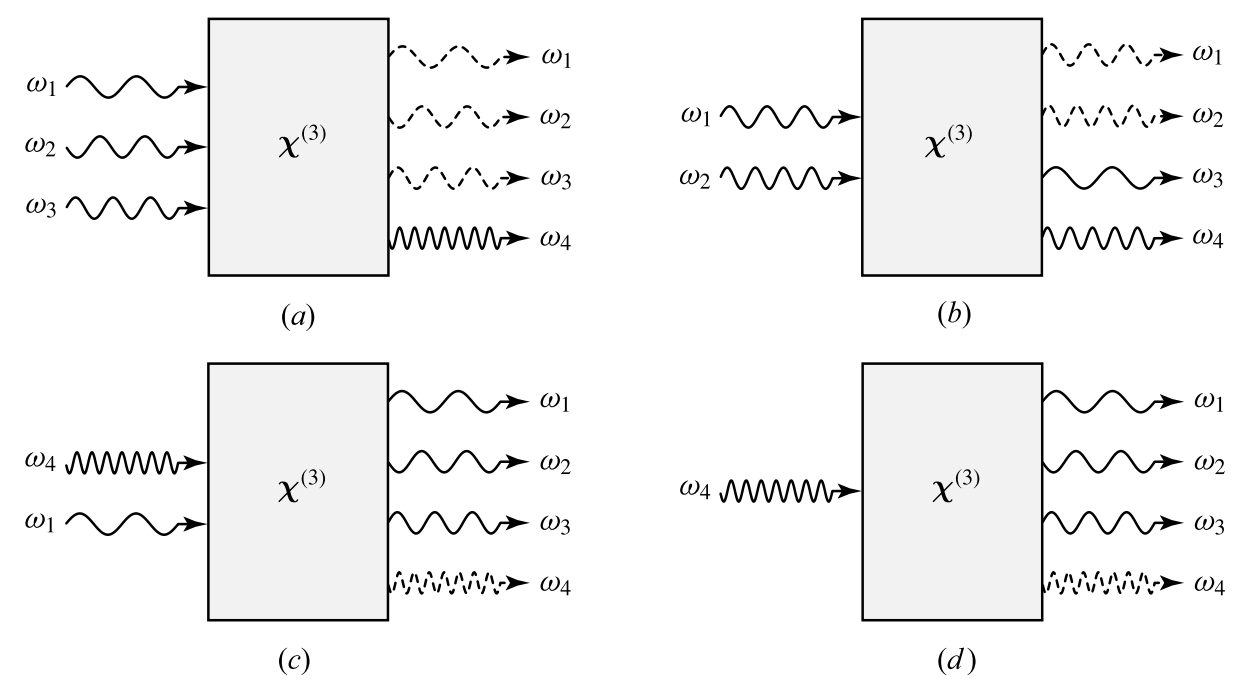Figure 9-4. Third-order parametric frequency conversion processes. (a) $$\omega_1+\omega_2+\omega_3\rightarrow\omega_4$$. (b) $$\omega_1+\omega_2\rightarrow\omega_3+\omega_4$$. (c) $$\omega_4-\omega_1\rightarrow\omega_2+\omega_3$$. (d) $$\omega_4\rightarrow\omega_1+\omega_2+\omega_3$$.

In a scenario similar to sum-frequency generation, three photons at frequencies $$\omega_1$$, $$\omega_2$$, and $$\omega_3$$ combine to generate a photon at a higher frequency $$\omega_4=\omega_1+\omega_2+\omega_3$$, as shown in Figure 9-4(a).

In another scenario, shown in Figure 9-4(b), two photons at $$\omega_1$$ and $$\omega_2$$ combine to generate two other photons at $$\omega_3$$ and $$\omega_4$$.

In yet another scenario, which is similar to difference-frequency generation, shown in Figure 9-4(c), one photon at $$\omega_4$$ breaks into three photons at $$\omega_1$$, $$\omega_2$$, and $$\omega_3$$ through the interaction of the wave at $$\omega_4$$ with another wave at a lower frequency, say $$\omega_1$$.

In a process similar to parametric generation, it is also possible for a photon at $$\omega_4$$ to break into three photons at $$\omega_1$$, $$\omega_2$$, and $$\omega_3$$ without an input at any of the lower frequencies, as shown in Figure 9-4(d).

Third-harmonic generation is simply the degenerate case of the first scenario for $$\omega_1=\omega_2=\omega_3=\omega$$ and $$\omega_4=3\omega$$.

Other partially degenerate cases exist also. For example, for any of the three scenarios shown in Figure 9-4, it is possible that $$\omega_1=\omega_2=\omega$$ but $$\omega_3\ne\omega$$ and $$\omega_3\ne\omega_4$$.

The parametric frequency conversion processes are not the only third-order nonlinear processes that result in optical frequency conversion. Among the nonparametric processes, stimulated Raman scattering and stimulated Brillouin scattering also lead to optical frequency conversion.

However, being nonparametric, these processes are connected to the intrinsic resonances of the medium and are dependent on the initial state of the material.

If the material is originally in the ground state of the relevant transition, annihilation of a photon at the pump frequency, $$\omega_\text{p}$$, of the incident optical wave creates an excitation in the material and a photon at a down-shifted Stokes frequency $$\omega_\text{S}=\omega_\text{p}-\Omega$$, as illustrated in Figure 9-5(a) below.

If the material is originally in an excited state, it is possible to create a photon at an up-shifted anti-Stokes frequency $$\omega_\text{AS}=\omega_\text{p}+\Omega$$ while the material simultaneously makes a transition from the excited state to the ground state, as illustrated in Figure 9-5(b) below.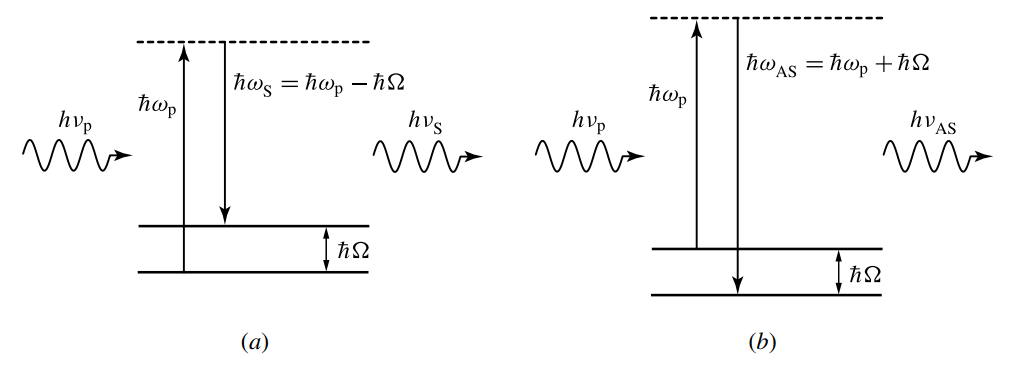Figure 9-5. (a) Stokes and (b) anti-Stokes transitions for stimulated Raman scattering

The Stokes susceptibility is $${\boldsymbol{\chi}^{(3)}}''(\omega_\text{S}=\omega_\text{S}+\omega_\text{p}-\omega_\text{p})$$, whereas the anti-Stokes susceptibility is $${\boldsymbol{\chi}^{(3)}}''(\omega_\text{AS}=\omega_\text{AS}+\omega_\text{p}-\omega_\text{p})$$. The amount of frequency shift, $$\Omega=\omega_\text{p}-\omega_\text{S}=\omega_\text{AS}-\omega_\text{p}$$, is determined by the excitation responsible for a given process and is a characteristic of the material.

The fundamental difference between a Raman process and Brillouin process is the mode of excitation in the material that participates in the interaction. In stimulated Raman scattering, the interaction is associated with the excitation at the molecular or atomic level, such as the optical phonons of a medium or the vibrational modes of molecules. In stimulated Brillouin scattering, the interaction is associated with the long-range excitation characterized by the acoustic phonons, or the acoustic wave, of a medium.

Other third-order processes listed in Table 9-5 do not cause optical frequency conversion but have the characteristic of inducing field-dependent changes in the optical properties of a material. These processes are characterized by either the real or imaginary part of a susceptibility of the form $$\boldsymbol{\chi}^{(3)}(\omega=\omega+\omega'-\omega')$$. When $$\omega'=\omega$$, there can be only one beam in the interaction, as illustrated in Figure 9-6(a), but here can also be two physically distinguishable beams of the same frequency, as illustrated in Figure 9-6(b). When $$\omega'\ne\omega$$, there are always two optical beams in the interaction, as illustrated in Figure 9-6(c).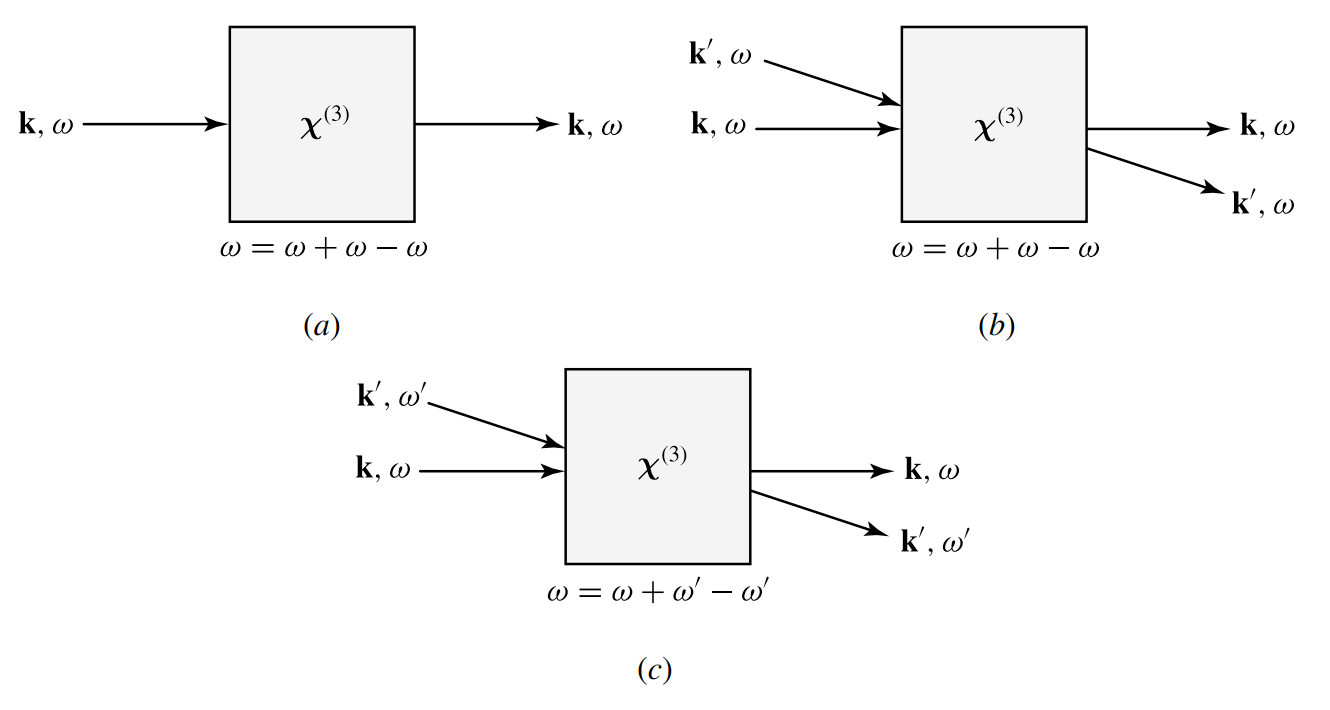Figure 9-6. Third-order processes for field-induced susceptibility changes: (a) one-beam interaction, (b) interaction of two beams of the same frequency, and (c) interaction of two beams of different frequencies.

In the case of one-beam interaction, we find that

$\tag{9-43}P_i^{(3)}(\omega)=3\epsilon_0\sum_{j,k,l}\chi_{ijkl}^{(3)}(\omega=\omega+\omega-\omega)E_j(\omega)E_k(\omega)E_l^*(\omega)$

using (9-22) [refer to the optical nonlinearity tutorial] and the intrinsic permutation symmetry.

In the case of two-beam interaction, for either $$\omega'=\omega$$ or $$\omega'\ne\omega$$, we have

\tag{9-44}\begin{align}P_i^{(3)}(\omega)=&3\epsilon_0\sum_{j,k,l}\chi_{ijkl}^{(3)}(\omega=\omega+\omega-\omega)E_j(\omega)E_k(\omega)E_l^*(\omega)\\&+6\epsilon_0\sum_{j,k,l}\chi_{ijkl}^{(3)}(\omega=\omega+\omega'-\omega')E_j(\omega)E_k(\omega')E_l^*(\omega')\end{align}

and a similar expression for $$P_i^{(3)}(\omega')$$ in terms of $$\chi_{ijkl}^{(3)}(\omega'=\omega'+\omega'-\omega')$$ and $$\chi_{ijkl}^{(3)}(\omega'=\omega'+\omega-\omega)$$.

By identifying the total polarization at the frequency $$\omega$$ as $$P_i(\omega)=P_i^{(1)}(\omega)+P_i^{(3)}(\omega)$$, we find that the total field-dependent permittivity tensor can be expressed as

$\tag{9-45}\epsilon_{ij}(\omega,\mathbf{E})=\epsilon_{ij}(\omega)+\Delta\epsilon_{ij}(\omega,\mathbf{E})$

where $$\epsilon_{ij}(\omega)=\epsilon_0\left[1+\chi_{ij}^{(1)}(\omega)\right]$$ represents the field-dependent linear permittivity tensor of the medium and $$\Delta\epsilon_{ij}(\omega,\mathbf{E})$$ accounts for the field-dependent change induced by nonlinear optical interaction.

For one-beam interaction,

$\tag{9-46}\Delta\epsilon_{ij}(\omega,\mathbf{E})=3\epsilon_0\sum_{k,l}\chi_{ijkl}^{(3)}(\omega=\omega+\omega-\omega)E_k(\omega)E_l^*(\omega)$

For two-beam interaction,

\tag{9-47}\begin{align}\Delta\epsilon_{ij}(\omega,\mathbf{E})=&3\epsilon_0\sum_{k,l}\chi_{ijkl}^{(3)}(\omega=\omega+\omega-\omega)E_k(\omega)E_l^*(\omega)\\&+6\epsilon_0\sum_{k,l}\chi_{ijkl}^{(3)}(\omega=\omega+\omega'-\omega')E_k(\omega')E_l^*(\omega')\end{align}

The field-dependent permittivity of the form described here is the basis of many nonlinear optical phenomena that have important practical applications.

The nonlinear process discussed here generally leads to an optical-field-induced birefringence because $$\Delta\epsilon_{ij}$$ is a tensor. The simplest case involves a single linearly polarized optical wave in an isotropic medium with the optical field polarized in any fixed direction, or in a cubic crystal with the optical field polarized along one of the crystal axes. Then $$\mathbf{P}^{(3)}$$ is parallel to $$\mathbf{E}$$ of the optical field, and the only susceptibility element that contributes to this type of interaction is $$\chi_{1111}^{(3)}(\omega=\omega+\omega-\omega)$$. Therefore, the permittivity seen by the optical field is

\tag{9-48}\begin{align}\epsilon(\omega,\mathbf{E})&=\epsilon(\omega)+3\epsilon_0\chi_{1111}^{(3)}|E(\omega)|^2\\&=\epsilon(\omega)+\frac{3\chi_{1111}^{(3)}}{2cn_0}I(\omega)\end{align}

where $$n_0$$ is the linear refractive index of the medium and $$I(\omega)$$ is the intensity of the optical beam. We see from this relation that the real part of $$\chi_{1111}^{(3)}(\omega=\omega+\omega-\omega)$$ leads to the following intensity-dependent index of refraction:

$\tag{9-49}n=n_0+n_2I(\omega)$

where

$\tag{9-50}n_2=\frac{3\chi_{1111}^{(3)'} }{4c\epsilon_0n_0^2}(\text{m}^2\text{W}^{-1})$

This intensity-dependent index of refraction represents the simplest case of the optical Kerr effect. Depending on the material properties and the experimental conditions, it leads to the phenomena of self-phase modulation, self focusing, and self defocusing.

The value of $$n_2$$ for a given material varies with optical wavelength, impurities, and temperature. In particular, it can be significantly enhanced by transition resonances in a manner like the resonant enhancement of the linear refractive index.

Its value also depends on its response speed because $$n_2$$ of a given material can be contributed by many different physical mechanisms that have different relaxation times. For example, the value of $$n_2$$ at room temperature of a semiconductor, such as GaAs or AlGaAs, can range from the order of $$1\times10^{-17}\text{ m}^2\text{W}^{-1}$$ for wavelengths far away from the absorption bandgap to the order of $$2\times10^{-10}\text{ m}^2\text{W}^{-1}$$ for wavelengths near the bandgap with exciton enhancement, and then further to the order of $$1\times10^{-8}\text{ m}^2\text{W}^{-1}$$ with exciton enhancement near the band edge in GaAs/AlGaAs quantum wells.

Example 9-5

Silica glass has an electronically contributed nonlinear susceptibility of $$\chi_{1111}^{(3)}(\omega=\omega+\omega-\omega)=1.8\times10^{-22}\text{ m}^2\text{V}^{-2}$$ that causes an intensity-dependent index change in optical fibers. Its linear refractive index is $$n_0\approx1.45$$ in the visible and near infrared spectral regions of interest for most applications of optical fibers. Find the value of $$n_2$$ for silica fibers. For an ultrashort optical pulse that has a pulsewidth on the order or picoseconds or femtoseconds, the peak power can easily be a few kilowatts. Take a femtosecond pulse of a 10 kW peak power that propagates in a fiber of a 10 μm core diameter. What is the optical-field-induced index change seen by this pulse?

Using (9-50), we find that

$n_2=\frac{3\times1.8\times10^{-22}}{4\times3\times10^8\times8.85\times10^{-12}\times1.45^2}\text{ m}^2\text{ W}^{-1}=2.4\times10^{-20}\text{ m}^2\text{ W}^{-1}$

Therefore, $$n_2=2.4\times10^{-20}\text{ m}^2\text{ W}^{-1}$$ for silica fibers. The optical-field-induced index change seen by the pulse is

$\Delta{n}=n_2I=2.4\times10^{-20}\times\frac{10\times10^3}{\pi\times(10\times10^{-6}/2)^2}=3\times10^{-6}$

We see that the optical-field-induced index change is very small even for a pulse of 10 kW peak power confined in a very small fiber core to reach a very high intensity. Nevertheless, this small index change can have very significant effects on the characteristics of the optical pulse through the process of self-phase modulation, and on other pulses through cross-phase modulation. It is the root of such fascinating phenomena as optical solitons and pulse spectral broadening. The value of $$n_2$$ for optical fibers varies with the dopants in the fiber and with the optical wavelength. In the literature, the value of $$n_2=3.2\times10^{-20}\text{ m}^2\text{ W}^{-1}$$ is often quoted for optical fibers. In Er-doped fibers, the value of $$n_2$$ at a resonance wavelength of 980 nm can be increased to the order of $$1$$ to $$3\times10^{-15}\text{ m}^2\text{ W}^{-1}$$, depending on the doping concentration, due to resonance enhancement.

From (9-48), we also see that the imaginary part of the total susceptibility is

$\tag{9-51}\chi''=\chi^{(1)''}+\frac{3\chi_{1111}^{(3)''}}{2cn_0}I(\omega)$

Therefore, the imaginary part of $$\chi_{1111}^{(3)}(\omega=\omega+\omega-\omega)$$ leads to an intensity-dependent change in the loss or gain of a medium.

As a general rule, the sign of $$\chi_{1111}^{(3)''}(\omega=\omega+\omega-\omega)$$ that is contributed by a single-photon transition of a resonance frequency at or near $$\omega$$ is always the opposite of that of $$\chi^{(1)''}(\omega)$$.

When $$\chi^{(1)''}\gt0$$, the medium has a linear loss but $$\chi^{(3)''}$$ is negative and contributes to an intensity-dependent reduction of the loss, resulting in absorption saturation.

When $$\chi^{(1)''}\lt0$$, the medium has a gain. Then, $$\chi^{(3)''}$$ is positive and causes intensity-dependent gain saturation.

The characteristics of a nonparametric third-order process are determined by the resonant transition that is responsible for the process.

In Table 9-5, we see that the susceptibility for two-photon absorption has the same form as that for stimulated Raman scattering. However, the resonance frequency of the transition for stimulated Raman scattering is the difference $$\omega_\text{p}-\omega_\text{S}$$, whereas that for two-photon absorption is the sum $$\omega_1+\omega_2$$, as shown in figure 9-7(a) below.

In the special case when $$\omega_1=\omega_2=\omega$$, both the susceptibility for two-photon absorption and that for absorption saturation or gain saturation have the form $$\boldsymbol{\chi}^{(3)''}(\omega=\omega+\omega-\omega)$$. The difference between them is that the nonlinear susceptibility responsible for absorption saturation or gain saturation is resonant at $$\omega$$ but that for two-photon absorption is resonant at $$2\omega$$, as shown in figure 9-7(b) below.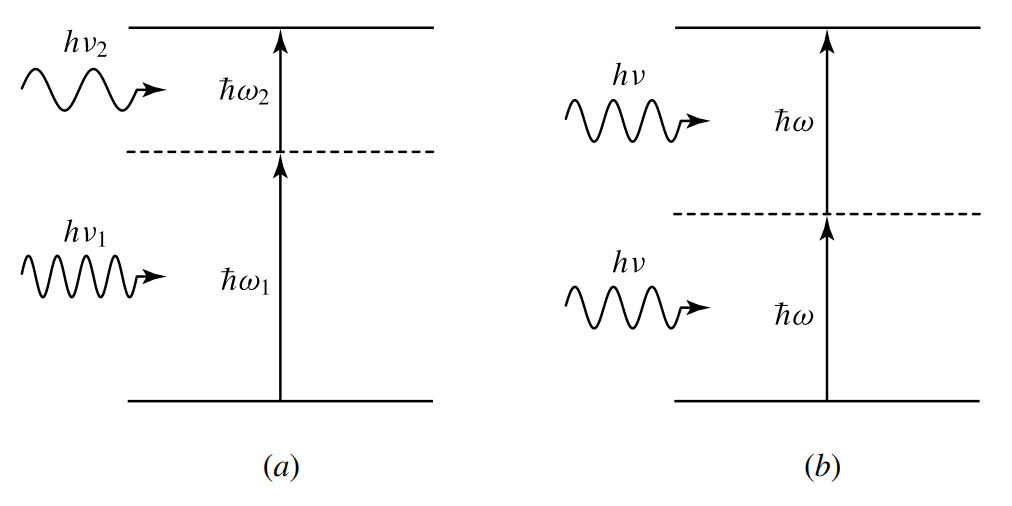Figure 9-7. Resonant transitions for two-photon absorption at (a) $$\omega_1+\omega_2$$ and $$2\omega$$.

A nonlinear process that characterizes the interaction of four optical waves is generally referred to as four-wave mixing. In nondegenerate four-wave mixing, all four optical waves have different frequencies. The process becomes partially degenerate if there are only two or three distinct frequencies. In degenerate four-wave mixing, all of the participating waves have the same frequency. With the exception of the electro-optic Kerr effect, all of the third-order nonlinear processes can be described as four-wave mixing processes.

The next part continues with the coupled-wave analysis of nonlinear optical interactions tutorial